MODEL Statement

Subsections:

MODEL <transform(dependents </ t-options>)><transform(dependents </ t-options>)=> transform(independents </ t-options>)<transform(independents </ t-options>)…> </ a-options> ;

The MODEL statement specifies the dependent and independent variables (dependents and independents, respectively) and specifies the transformation (transform) to apply to each variable. Only one MODEL statement can appear in PROC TRANSREG. The t-options are transformation options, and the a-options are algorithm options. The t-options provide details for the transformation; these depend on the transform chosen. The t-options are listed after a slash in the parentheses that enclose the variable list (either dependents or independents). The a-options control the algorithm used, details of iteration, details of how the intercept and coded variables are generated, and displayed output details. The a-options are listed after the entire model specification (the dependents, independents, transformations, and t-options) and after a slash. You can also specify the algorithm options in the PROC TRANSREG statement. When you specify the DESIGN o-option, dependents and an equal sign are not required. The operators *, |, and @ from the GLM procedure are available for interactions with the CLASS expansion and the IDENTITY transformation. They are used as follows:

      Class(a * b ...
c | d ...
e | f ... @ n)
Identity(a * b ...
c | d ...
e | f ... @ n)


In addition, transformations and spline expansions can be crossed with classification variables as follows:

transform(var) * class(group) transform(var) | class(group)

See the section Types of Effects in Chapter 44: The GLM Procedure, for a description of the @, *, and | operators and see the section Model Statement Usage for information about how to use these operators in PROC TRANSREG. Note that nesting is not implemented in PROC TRANSREG.

The next three sections discuss the transformations available (transforms) (see the section Families of Transformations), the transformation options (t-options) (see the section Transformation Options (t-options)), and the algorithm options (a-options) (see the section Algorithm Options (a-options)).

Families of Transformations

In the MODEL statement, transform specifies a transformation in one of the following five families:

Variable expansions

preprocess the specified variables, replacing them with more variables.

Nonoptimal transformations

preprocess the specified variables, replacing each one with a single new nonoptimal, nonlinear transformation.

Nonlinear fit transformations

preprocess the specified variable, replacing it with a smooth transformation, fitting one or more nonlinear functions through a scatter plot.

Optimal transformations

replace the specified variables with new, iteratively derived optimal transformation variables that fit the specified model better than the original variable (except for contrived cases where the transformation fits the model exactly as well as the original variable).

Other transformations

are the IDENTITY and SSPLINE transformations. These do not fit into the preceding categories.

The transformations and expansions listed in Table 101.2 are available in the MODEL statement.

Table 101.2: Transformation Families

Transformation

Description

Variable Expansions

B-spline basis

set of coded variables

elliptical response surface

circular response surface & PREFMAP

piecewise polynomial basis

Nonoptimal Transformations

inverse trigonometric sine

exponential

logarithm

logit

raises variables to specified power

transforms to ranks

Nonlinear Fit Transformations

Box-Cox

penalized B-splines

noniterative smoothing spline

Optimal Transformations

linear

monotonic, ties preserved

monotonic B-spline

optimal scoring

B-spline

monotonic, ties not preserved

Other Transformations

identity, no transformation

iterative smoothing spline

You can use any transformation with either dependent or independent variables (except the SMOOTH and PBSPLINE transformations, which can be used only with independent variables, and BOXCOX, which can be used only with dependent variables). However, the variable expansions are usually more appropriate for independent variables.

The transform is followed by a variable (or list of variables) enclosed in parentheses. Here is an example:

model log(y) = class(x);


This example finds a LOG transformation of y and performs a CLASS expansion of x. Optionally, depending on the transform, the parentheses can also contain t-options, which follow the variables and a slash. Here is an example:

model identity(y) = spline(x1 x2 / nknots=3);


The preceding statement finds SPLINE transformations of x1 and x2. The NKNOTS= t-option used with the SPLINE transformation specifies three knots. The identity(y) transformation specifies that y is not to be transformed.

The rest of this section provides syntax details for members of the five families of transformations listed at the beginning of this section. The t-options are discussed in the section Transformation Options (t-options).

Variable Expansions

PROC TRANSREG performs variable expansions before iteration begins. Variable expansions expand the original variables into a typically larger set of new variables. The original variables are those that are listed in parentheses after transform, and they are sometimes referred to by the name of the transform. For example, in CLASS(x1 x2), x1 and x2 are sometimes referred to as CLASS expansion variables or simply CLASS variables, and the expanded variables are referred to as coded or sometimes dummy variables. Similarly, in POINT(Dim1 Dim2), Dim1 and Dim2 are sometimes referred to as POINT variables.

The resulting variables are not transformed by the iterative algorithms after the initial preprocessing. Observations with missing values for these types of variables are excluded from the analysis.

The POINT, EPOINT, and QPOINT variable expansions are used in preference mapping analyses (also called PREFMAP, external unfolding, ideal point regression) (Carroll, 1972) and for response surface regressions. These three expansions create circular, elliptical, and quadratic response or preference surfaces (see the section Point Models and Example 101.6). The CLASS variable expansion is used for main-effects ANOVA.

The following list provides syntax and details for the variable expansion transforms.

BSPLINE
BSP

expands each variable to a B-spline basis. You can specify the DEGREE=, KNOTS=, NKNOTS=, and EVENLY= t-options with the BSPLINE expansion. When DEGREE=n (3 by default) with k knots (0 by default),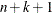variables are created. In addition, the original variable appears in the OUT= data set before the ID variables. For example, bspline(x) expands x into x_0 x_1 x_2 x_3 and outputs x as well. The x_: variables contain the B-spline basis vectors (which are the same basis vectors that the SPLINE and MSPLINE transformations use internally). The columns of the BSPLINE expansion sum to a column of ones, so an implicit intercept model is fit when the BSPLINE expansion is specified. If you specify the BSPLINE expansion for more than one variable, the model is less than full rank. Variables specified in a BSPLINE expansion must be numeric, and they are typically continuous. See the sections SPLINE and MSPLINE Transformations and SPLINE, BSPLINE, and PSPLINE Comparisons for more information about B-splines.

CLASS
CLA

expands the variables to a set of coded or dummy variables. PROC TRANSREG uses the values of the formatted variables to determine class membership. The specification class(x1 x2) fits a simple main-effects model, class(x1 | x2) fits a main-effects and interactions model, and class(x1|x2|x3|x4@2 x1*x2*x3) fits a model with all main effects, all two-way interactions, and one three-way interaction. Variables specified with the CLASS expansion can be either character or numeric; numeric variables should be discrete. See the section ANOVA Codings for more information about CLASS variables. See the section Model Statement Usage for information about how to use the operators @, *, and | in PROC TRANSREG.

EPOINT
EPO

expands the variables for an elliptical response surface regression or for an elliptical ideal point regression. Specify the COORDINATES o-option to output PREFMAP ideal elliptical point model coordinates to the OUT= data set. Each axis of the ellipse (or ellipsoid) is oriented in the same direction as one of the variables. The EPOINT expansion creates a new variable for each original variable. The value of each new variable is the square of each observed value for the corresponding original variable. The regression analysis then uses both sets of variables (original and squared). Variables specified with the EPOINT expansion must be numeric, and they are typically continuous. See the section Point Models and Example 101.6 for more information about point models.

POINT
POI

expands the variables for a circular response surface regression or for a circular ideal point regression. Specify the COORDINATES o-option to output PREFMAP ideal point model coordinates to the OUT= data set. The POINT expansion creates a new variable having a value for each observation that is the sum of squares of all the POINT variables. This new variable is added to the set of variables and is used in the regression analysis. For more information about ideal point regression, see Carroll (1972). Variables specified with the POINT expansion must be numeric, and they are typically continuous. See the section Point Models and Example 101.6 for more information about point models.

PSPLINE
PSP

expands each variable to a piecewise polynomial basis. You can specify the DEGREE=, KNOTS=, NKNOTS=, and EVENLY t-options with PSPLINE. When DEGREE=n (3 by default) with k knots (0 by default),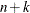variables are created. In addition, the original variable appears in the OUT= data set before the ID variables. For example, pspline(x / nknots=1) expands x into x_1 x_2 x_3 x_4 and outputs x as well. Unlike BSPLINE, an intercept is not implicit in the columns of PSPLINE. Variables specified with the PSPLINE expansion must be numeric, and they are typically continuous. See the sections SPLINE, BSPLINE, and PSPLINE Comparisons and Using Splines and Knots for more information about splines. Also see Smith (1979) for a good introduction to piecewise polynomial splines.

QPOINT
QPO

expands the variables for a quadratic response surface regression or for a quadratic ideal point regression. Specify the COORDINATES o-option to output PREFMAP quadratic ideal point model coordinates to the OUT= data set. For m QPOINT variables,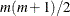new variables are created containing the squares and crossproducts of the original variables. The regression analysis uses both sets (original and crossed). Variables specified with the QPOINT expansion must be numeric, and they are typically continuous. See the section Point Models and Example 101.6 for more information about point models.

Nonoptimal Transformations

The nonoptimal transformations, like the variable expansions, are computed before the iterative algorithm begins. Nonoptimal transformations create a single new transformed variable that replaces the original variable. The new variable is not transformed by the subsequent iterative algorithms (except for a possible linear transformation with missing value estimation). The following list provides syntax and details for nonoptimal variable transformations.

ARSIN
ARS

finds an inverse trigonometric sine transformation. Variables specified in the ARSIN transform must be numeric and in the interval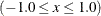, and they are typically continuous.

EXP

exponentiates variables (x is transformed to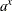). To specify the value of a, use the PARAMETER= t-option. By default, a is the mathematical constant e = 2.718…. Variables specified with the EXP transform must be numeric, and they are typically continuous.

LOG

transforms variables to logarithms (x is transformed to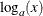). To specify the base of the logarithm, use the PARAMETER= t-option. The default is a natural logarithm with base e = 2.718…. Variables specified with the LOG transform must be numeric and positive, and they are typically continuous.

LOGIT

finds a logit transformation on the variables. The logit of x is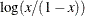. Unlike other transformations, LOGIT does not have a three-letter abbreviation. Variables specified with the LOGIT transform must be numeric and in the interval (0.0 < x < 1.0), and they are typically continuous.

POWER
POW

raises variables to a specified power (x is transformed to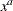). You must specify the power parameter a by specifying the PARAMETER= t-option following the variables. Here is an example:

power(variable / parameter=number)


You can use POWER for squaring variables (PARAMETER=2), reciprocal transformations (PARAMETER=–1), square roots (PARAMETER=0.5), and so on. Variables specified with the POWER transform must be numeric, and they are typically continuous.

RANK
RAN

transforms variables to ranks. Ranks are averaged within ties. The smallest input value is assigned the smallest rank. Variables specified in the RANK transform must be numeric.

Nonlinear Fit Transformations

Nonlinear fit transformations, like nonoptimal transformations, are computed before the iterative algorithm begins. Nonlinear fit transformations create a single new transformed variable that replaces the original variable and provides one or more smooth functions through a scatter plot. The new variable is not transformed by the subsequent iterative algorithms. The nonlinear fit transformations, unlike the nonoptimal transformations, use information in the other variables in the model to find the transformations. The nonlinear fit transformations, unlike the optimal transformations, do not minimize a squared-error criterion. The following list provides syntax and details for nonoptimal variable transformations.

BOXCOX
BOX

finds a Box-Cox (1964) transformation of the specified variables. The BOXCOX transformation can be used only with dependent variables. The ALPHA=, CLL=, CONVENIENT, GEOMETRICMEAN, LAMBDA=, and PARAMETER= t-options can be used with the BOXCOX transformation. Variables specified in the BOXCOX transform must be numeric, and they are typically continuous. See the section Box-Cox Transformations and Example 101.2 for more information about Box-Cox transformations.

PBSPLINE
PBS

is a noniterative penalized B-spline transformation (Eilers and Marx, 1996). The PBSPLINE transformation can be used only with independent variables. By default with PBSPLINE, a cubic spline is fit with 100 evenly spaced knots, three evenly spaced exterior knots, and a difference matrix of order three (DEGREE=3 NKNOTS=100 EVENLY=3 PARAMETER=3). Variables specified in the PBSPLINE transform must be numeric, and they are typically continuous. See the section Penalized B-Splines and Example 101.3 for more information about penalized B-splines.

SMOOTH
SMO

is a noniterative smoothing spline transformation (Reinsch, 1967). You can specify the smoothing parameter with either the SM= or the PARAMETER= t-option. The default smoothing parameter is SM=0. The SMOOTH transformation can be used only with independent variables. Variables specified with the SMOOTH transform must be numeric, and they are typically continuous. See the sections Smoothing Splines and Smoothing Splines Changes and Enhancements for more information about smoothing splines.

Optimal Transformations

Optimal transformations are iteratively derived. Missing values for these types of variables can be optimally estimated (see the section Missing Values). The following list provides syntax and details for optimal transformations.

LINEAR
LIN

finds an optimal linear transformation of each variable. For variables with no missing values, the transformed variable is the same as the original variable. For variables with missing values, the transformed nonmissing values have a different scale and origin than the original values. Variables specified in the LINEAR transform must be numeric. See the section OPSCORE, MONOTONE, UNTIE, and LINEAR Transformations for more information about optimal scaling.

MONOTONE
MON

finds a monotonic transformation of each variable, with the restriction that ties are preserved. The Kruskal (1964) secondary least squares monotonic transformation is used. This transformation weakly preserves order and category membership (ties). Variables specified with the MONOTONE transform must be numeric, and they are typically discrete. See the section OPSCORE, MONOTONE, UNTIE, and LINEAR Transformations for more information about optimal scaling.

MSPLINE
MSP

finds a monotonically increasing B-spline transformation with monotonic coefficients (de Boor, 1978; de Leeuw, 1986) of each variable. You can specify the DEGREE=, KNOTS=, NKNOTS=, and EVENLY= t-options with MSPLINE. By default, PROC TRANSREG fits a quadratic spline with no knots. Variables specified with the MSPLINE transform must be numeric, and they are typically continuous. See the section SPLINE and MSPLINE Transformations for more information about monotone splines.

OPSCORE
OPS

finds an optimal scoring of each variable. The OPSCORE transformation assigns scores to each class (level) of the variable. The Fisher (1938) optimal scoring method is used. Variables specified with the OPSCORE transform can be either character or numeric; numeric variables should be discrete. See the sections Character OPSCORE Variables and OPSCORE, MONOTONE, UNTIE, and LINEAR Transformations for more information about optimal scaling.

SPLINE
SPL

finds a B-spline transformation (de Boor, 1978) of each variable. By default, PROC TRANSREG fits a cubic spline with no knots. You can specify the DEGREE=, KNOTS=, NKNOTS=, and EVENLY= t-options with SPLINE. Variables specified with the SPLINE transform must be numeric, and they are typically continuous. See the sections SPLINE and MSPLINE Transformations, Specifying the Number of Knots, and SPLINE, BSPLINE, and PSPLINE Comparisons, and Using Splines and Knots for more information about splines.

UNTIE
UNT

finds a monotonic transformation of each variable without the restriction that ties are preserved. PROC TRANSREG uses the Kruskal (1964) primary least squares monotonic transformation method. This transformation weakly preserves order but not category membership (it might untie some previously tied values). Variables specified with the UNTIE transform must be numeric, and they are typically discrete. See the section OPSCORE, MONOTONE, UNTIE, and LINEAR Transformations for more information about optimal scaling.

Other Transformations

IDENTITY
IDE

specifies variables that are not changed by the iterations. Typically, the IDENTITY transformation is used with a simple variable list, such as identity(x1-x5). However, you can also specify interaction terms. For example, identity(x1 | x2) creates x1, x2, and the product x1*x2; and identity(x1 | x2 | x3) creates x1, x2, x1*x2, x3, x1*x3, x2*x3, and x1*x2*x3. See the section Model Statement Usage for information about how to use the operators @, *, and | in PROC TRANSREG. Variables specified in the IDENTITY transform must be numeric.

The IDENTITY transformation is used for variables when no transformation and no missing data estimation are desired. However, the REFLECT t-option, the ADDITIVE a-option, and the TSTANDARD=Z, and TSTANDARD=CENTER options can linearly transform all variables, including IDENTITY variables, after the iterations. Observations with missing values in IDENTITY variables are excluded from the analysis, and no optimal scores are computed for missing values in IDENTITY variables.

SSPLINE
SSP

finds an iterative smoothing spline transformation of each variable. The SSPLINE transformation does not generally minimize squared error. You can specify the smoothing parameter with either the SM= t-option or the PARAMETER= t-option. The default smoothing parameter is SM=0. Variables specified with the SSPLINE transform must be numeric, and they are typically continuous.

Transformation Options (t-options)

If you use a nonoptimal, nonlinear fit, optimal, or other transformation, you can use t-options, which specify additional details of the transformation. The t-options are specified within the parentheses that enclose variables and are listed after a slash. You can use t-options with both the dependent and the independent variables. Here is an example of using just one t-option:

proc transreg;
model identity(y)=spline(x / nknots=3);
output;
run;


The preceding statements find an optimal variable transformation (SPLINE) of the independent variable, and they use a t-option to specify the number of knots (NKNOTS=). The following is a more complex example:

proc transreg;
model mspline(y / nknots=3)=class(x1 x2 / effects);
output;
run;


These statements find a monotone spline transformation (MSPLINE with three knots) of the dependent variable and perform a CLASS expansion with effects coding of the independents.

Table 101.3 summarizes the t-options available in the MODEL statement.

Table 101.3: Transformation Options

Option

Description

Nonoptimal Transformation

Uses original mean and variance

Parameter Specification

Specifies miscellaneous parameters

Specifies smoothing parameter

Penalized B-Spline

Uses Akaike’s information criterion

Uses corrected AIC

Uses cross validation criterion

Uses generalized cross validation criterion

Specifies smoothing parameter list or range

Specifies a LAMBDA= range, not a list

Uses Schwarz’s Bayesian criterion

Spline

Specifies the degree of the spline

Spaces the knots evenly

Specifies exterior knots

Specifies the interior knots or break points

Creates n knots

CLASS Variable

Specifies CLASS coded variable name prefix

Specifies a deviations-from-means coding

Specifies a deviations-from-means coding

Specifies CLASS coded variable label prefix

Specifies order of CLASS variable levels

Specifies an orthogonal-contrast coding

Specifies CLASS coded variable label separators

Specifies a standardized-orthogonal coding

Controls reference levels

Box-Cox

Specifies confidence interval alpha

Specifies convenient lambda list

Uses a convenient lambda

GEOMETRICMEAN

Scales transformation using geometric mean

Specifies power parameter list

Other t-options

Specifies operations occur after the expansion

Specifies center before the analysis begins

Renames variables

Reflects the variable around the mean

Specifies transformation standardization

Standardizes before the analysis begins

The following sections discuss the t-options available for nonoptimal, nonlinear fit, optimal, and other transformations.

Nonoptimal Transformation t-options

ORIGINAL
ORI

matches the variable’s final mean and variance to the mean and variance of the original variable. By default, the mean and variance are based on the transformed values. The ORIGINAL t-option is available for all of the nonoptimal transformations.

Parameter t-options

PARAMETER=number
PAR=number

specifies the transformation parameter. The PARAMETER= t-option is available for the BOXCOX, EXP, LOG, POWER, SMOOTH, SSPLINE, and PBSPLINE transformations. For BOXCOX, the parameter is the value to add to each value of the variable before a Box-Cox transformation. For EXP, the parameter is the value to be exponentiated; for LOG, the parameter is the base value; and for POWER, the parameter is the power. For SMOOTH and SSPLINE, the parameter is the raw smoothing parameter. (See the SM= option for an alternative way to specify the smoothing parameter.) The default for the PARAMETER= t-option for the BOXCOX transformation is 0 and for the LOG and EXP transformations is e = 2.718…. The default parameter for SMOOTH and SSPLINE is computed from SM=0. For the POWER transformation, you must specify the PARAMETER= t-option; there is no default. For PBSPLINE, the parameter is the order of the difference matrix, which provides some control over the smoothness of the transformation. The default order parameter with PBSPLINE is the maximum of the DEGREE= t-option, and 1. With PBSPLINE, the default is DEGREE=3 and PARAMETER=3, which works well for most problems.

SM=n

specifies a smoothing parameter in the range 0 to 100, just like PROC GPLOT uses. For example, SM=50 in PROC TRANSREG is equivalent to I=SM50 in the SYMBOL statement with PROC GPLOT. You can specify the SM= t-option only with the SMOOTH and SSPLINE transformations. The smoothness of the function increases as the value of the smoothing parameter increases. By default, SM=0.

Spline t-options

The following t-options are available with the SPLINE, MSPLINE and PBSPLINE transformations and with the PSPLINE and BSPLINE expansions.

DEGREE=n
DEG=n

specifies the degree of the spline transformation. The degree must be a nonnegative integer. The defaults are DEGREE=3 for SPLINE, PSPLINE, and BSPLINE variables and DEGREE=2 for MSPLINE variables.

The polynomial degree should be a small integer, usually 0, 1, 2, or 3. Larger values are rarely useful. If you have any doubt as to what degree to specify, use the default.

EVENLY<=n>
EVE<=n>

is used with the NKNOTS= t-option to space the knots evenly. The differences between adjacent knots are constant.

If you specify NKNOTS=k and EVENLY, k knots are created at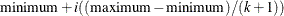for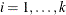. Here is an example:

spline(x / nknots=2 evenly)


When the variable x has a minimum of 4 and a maximum of 10, then the two interior knots are 6 and 8. Without the EVENLY t-option, the NKNOTS= t-option places knots at percentiles, so the knots are not evenly spaced. By default for the BSPLINE expansion and the SPLINE and MSPLINE transformations, the smaller exterior knots are all the same and all just a little smaller than the minimum. Similarly, by default, the larger exterior knots are all the same and all just a little larger than the maximum. However, if you specify EVENLY=n, then the n exterior knots are evenly spaced as well. The number of exterior knots must be greater than or equal to the degree. You can specify values larger than the degree when you want to interpolate slightly beyond the range or your data. The exterior knots must be less than the minimum or greater than the maximum; hence the knots across all sets are not precisely equally spaced. For example, with data ranging from 0 to 10, and with EVENLY=3 and NKNOTS=4, the first exterior knots are –4.000000000001, –2.000000000001, and –0.000000000001, the interior knots are 2, 4, 6, and 8, and the second exterior knots are 10.000000000001, 12.000000000001, and 14.000000000001.

With the BSPLINE and PSPLINE expansions and the SPLINE and MSPLINE transformations, evenly spaced knots are not the default. With the PBSPLINE transformation, evenly spaced interior and exterior knots are the default. If you want unevenly spaced knots with PBSPLINE, you must use the KNOTS= t-option.

EXKNOTS=number-list
EXK=number-list

specifies exterior knots for SPLINE and MSPLINE transformations and BSPLINE expansions. Usually, this t-option is not needed; PROC TRANSREG automatically picks suitable exterior knots. The only time you need to use this option is when you want to ensure that the exact same basis is used for different splines, such as when you apply coefficients from one spline transformation to a variable in a different data set (see the section Scoring Spline Variables).

Specify one or two values. If the minimum EXKNOTS= value is less than the minimum data value, it is used as the exterior knot. If the maximum EXKNOTS= value is greater than the maximum data value, it is used as the exterior knot. Otherwise these values are ignored. When EXKNOTS= is specified with the CENTER or Z t-options, the knots apply to the original variable, not to the centered or standardized variable.

The B-spline transformations and expansions use a knot list consisting of exterior knots (values just smaller than the minimum), the specified (interior) knots, and exterior knots (values just larger than the minimum). You can use the DETAIL a-option to see all of these knots. If you use different exterior knots, you get different but equivalent B-spline bases. You can specify exterior knots in either the KNOTS= or EXKNOTS= t-options; however, for the BSPLINE expansion, the KNOTS= t-option creates extra all-zero basis columns, whereas the EXKNOTS= t-option gives you the correct basis. See the EVENLY= t-option for an alternative way to specify exterior knots.

KNOTS=number-list |  n TO m BY p
KNO=number-list | n TO m BY p

specifies the interior knots or break points. By default, there are no knots. The first time you specify a value in the knot list, it indicates a discontinuity in the nth (from DEGREE=n) derivative of the transformation function at the value of the knot. The second mention of a value indicates a discontinuity in the (n – 1) derivative of the transformation function at the value of the knot. Knots can be repeated any number of times for decreasing smoothness at the break points, but the values in the knot list can never decrease.

You cannot use the KNOTS= t-option with the NKNOTS= t-option. You should keep the number of knots small (see the section Specifying the Number of Knots).

NKNOTS=n
NKN=n

creates n knots, the first at the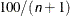percentile, the second at the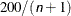percentile, and so on. Knots are always placed at data values; there is no interpolation. For example, if NKNOTS=3, knots are placed at the 25th percentile, the median, and the 75th percentile. You can use the EVENLY= t-option along with NKNOTS= to get evenly spaced knots. By default, with the BSPLINE and PSPLINE expansions and the SPLINE and MSPLINE transformations, NKNOTS=0. By default, with the PBSPLINE transformation, NKNOTS=100.

The value specified for the NKNOTS= t-option must be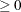.

You cannot use the NKNOTS= t-option with the KNOTS= t-option.

You should keep the number of knots small (see the section Specifying the Number of Knots).

Penalized B-Spline t-options

The following t-options are available with the PBSPLINE transformation.

AIC

specifies that the procedure should select the smoothing parameter,, that minimizes the (Akaike, 1973) information criterion (AIC). By default, the (AICC) criterion is minimized.

AICC

specifies that the procedure should select the smoothing parameter,, that minimizes the corrected Akaike information criterion (Hurvich, Simonoff, and Tsai, 1998). This is the default criterion unless the AIC, CV, GCV, or SBC t-option is specified.

CV

specifies that the procedure should select the smoothing parameter,, that minimizes the cross validation criterion (CV). By default, the (AICC) criterion is minimized.

GCV

specifies that the procedure should select the smoothing parameter,, that minimizes the generalized cross validation criterion (Craven and Wahba, 1979). By default, the (AICC) criterion is minimized.

LAMBDA=number-list
LAM=number-list

specifies a list of penalized B-spline smoothing parameters. By default, PROC TRANSREG considers lambdas in the range 0 to 1E6. Alternatively, you can specify the RANGE t-option with LAMBDA=, such as LAMBDA=1E3 1E5 RANGE, to only consider lambdas in a narrower range. Note that the algorithm might not actually evaluate the criterion at the minimum and maximum if it does not have to. In particular, it avoids evaluating the criterion at LAMBDA=0 (no smoothing) unless it is the only LAMBDA= value specified. You can also specify a list of lambdas, such as LAMBDA=1 TO 10, and the procedure selects the best lambda from the list. In all cases, the lambda that minimizes the specified criterion (or AICC by default) is chosen.

RANGE
RAN

specifies that the LAMBDA= t-option specifies two lambdas that define a range of values, from which an optimal lambda is selected. By default, PROC TRANSREG considers lambdas in the range 0 to 1E6.

SBC

specifies that the procedure should select the smoothing parameter,, that minimizes Schwarz’s Bayesian criterion (Schwarz, 1978; Judge et al., 1980). By default, the (AICC) criterion is minimized.

Class Variable t-options

CPREFIX=n | number-list
CPR=n | number-list

specifies the number of first characters of a CLASS expansion variable’s name to use in constructing names for coded variables. When you specify CPREFIX= as an a-option or an o-option, it specifies the default for all CLASS variables. When you specify CPREFIX= as a t-option, it overrides the default only for selected variables. A different CPREFIX= value can be specified for each CLASS variable by specifying the CPREFIX=number-list t-option, like the ZERO=formatted-value t-option.

DEVIATIONS
DEV

requests a deviations-from-means coding of CLASS variables. The coded design matrix has values of 0, 1, and –1 for reference levels. This coding is referred to as deviations-from-means, effects, center-point, or full-rank coding. For example, here is the coding for two-, three-, four-, and five-level factors:

 Number of Levels Two Three Four Five a 1 1 0 1 0 0 1 0 0 0 b -1 0 1 0 1 0 0 1 0 0 c -1 -1 0 0 1 0 0 1 0 d -1 -1 -1 0 0 0 1 e -1 -1 -1 -1

EFFECTS
EFF

See the DEVIATIONS t-option.

LPREFIX=n | number-list
LPR=n | number-list

specifies the number of first characters of a CLASS expansion variable’s label (or name if no label is specified) to use in constructing labels for the coded variables. When you specify LPREFIX= as an a-option or an o-option, it specifies the default for all CLASS variables. When you specify LPREFIX= as a t-option, it overrides the default only for selected variables. A different LPREFIX= value can be specified for each CLASS variable by specifying the LPREFIX=number-list t-option, like the ZERO=formatted-value t-option.

ORDER=DATA | FREQ | FORMATTED | INTERNAL
ORD=DAT | FRE | FOR | INT

specifies the order in which the CLASS variable levels are to be reported. The default is ORDER=INTERNAL. For ORDER=FORMATTED and ORDER=INTERNAL, the sort order is machine dependent. When you specify ORDER= as an a-option or an o-option, it specifies the default ordering for all CLASS variables. When you specify ORDER= as a t-option, it overrides the default ordering only for selected variables. You can specify a different ORDER= value for each CLASS specification.

ORTHOGONAL
ORT

requests an orthogonal-contrast coding of CLASS variables. For example, here is the orthogonal-contrast coding for two-, three-, four-, and five-level factors:

 Number of Levels Two Three Four Five a 1 1 -1 1 -1 -1 1 -1 -1 -1 b -1 0 2 0 2 -1 0 2 -1 -1 c -1 -1 0 0 3 0 0 3 -1 d -1 -1 -1 0 0 0 4 e -1 -1 -1 -1

The sum of the coded values within each column is zero, all columns within a factor are orthogonal, and the ith column represents a contrast between the ith level and the combination of all preceding levels and the last level. The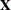matrix is orthogonal andis diagonal with this coding only if the experimental design is orthogonal.

SEPARATORS=’string-1’ <’string-2’>
SEP=’string-1’ <’string-2’>

specifies separators for creating CLASS expansion variable labels. By default, SEPARATORS=’ ’ ’ * ’ (blank and blank asterisk blank). When you specify SEPARATORS= as an a-option or an o-option, it specifies the default separators for all CLASS variables. When you specify SEPARATORS= as a t-option, it overrides the default only for selected variables. You can specify a different SEPARATORS= value for each CLASS specification.

STANDORTH
STA
ORTHEFFECT

requests a standardized-orthogonal coding of CLASS variables. For example, here is the standardized-orthogonal coding for two-, three-, four-, and five-level factors:

 Number of Levels Two Three Four Five a 1 1.22 -0.71 1.41 -0.82 -0.58 1.58 -0.91 -0.65 -0.50 b -1 0.00 1.41 0.00 1.63 -0.58 0.00 1.83 -0.65 -0.50 c -1.22 -0.71 0.00 0.00 1.73 0.00 0.00 1.94 -0.50 d -1.41 -0.82 -0.58 0.00 0.00 0.00 2.00 e -1.58 -0.91 -0.65 -0.50

The sum of the coded values within each column is zero, the sum of squares of the coded values within each column is equal to the number of levels, all columns within a factor are orthogonal, and the ith column represents a contrast between the ith level and the combination of all preceding levels and the last level. Thematrix is orthogonal andis diagonal (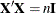, the number of observations times an identity matrix) with this coding only if the experimental design is orthogonal.

ZERO=FIRST | LAST | NONE | SUM
ZER=FIR | LAS | NON | SUM
ZERO=’formatted-value’ <’formatted-value’ …>

is used with CLASS variables. The default is ZERO=LAST.

The specification CLASS(variable / ZERO=FIRST) sets to missing the coded variable for the first of the sorted categories, implying a zero coefficient for that category.

The specification CLASS(variable / ZERO=LAST) sets to missing the coded variable for the last of the sorted categories, implying a zero coefficient for that category.

The specification CLASS(variable / ZERO=’formatted-value’) sets to missing the coded variable for the category with a formatted value that matches ’formatted-value’, implying a zero coefficient for that category. With ZERO=formatted-value, the first formatted value applies to the first variable in the specification, the second formatted value applies to the next variable that was not previously mentioned, and so on. For example, class(a a*b b b*c c / zero=’x’ ’y’ ’z’) specifies that the reference level for a is ’x’, for b is ’y’, and for c is ’z’. With ZERO=’formatted-value’, the procedure first looks for exact matches between the formatted values and the specified value. If none are found, leading blanks are stripped from both and the values are compared again. If zero or two or more matches are found, warnings are issued.

The specifications ZERO=FIRST, ZERO=LAST, and ZERO=’formatted-value’ are used for reference cell models. The Intercept parameter estimate is the marginal mean for the reference cell, and the other marginal means are obtained by adding the intercept to the coded variable coefficients.

The specification CLASS(variable / ZERO=NONE) sets to missing none of the coded variables. The columns of the expansion sum to a column of ones, so an implicit intercept model is fit. If you specify ZERO=NONE for more than one variable, the model is less than full rank. In the model model identity(y) = class(x / zero=none), the coefficients are cell means.

The specification CLASS(variable / ZERO=SUM) sets to missing none of the coded variables, and the coefficients for the coded variables created from the variable sum to 0. This creates a less-than-full-rank model, but the coefficients are uniquely determined due to the sum-to-zero constraint.

In the presence of iterative transformations, hypothesis tests for ZERO=NONE and ZERO=SUM levels are not exact; they are liberal because a model with an explicit intercept is fit inside the iterations. There is no provision for adjusting the transformations while setting to 0 a parameter that is redundant given the explicit intercept and the other parameters.

Box-Cox t-options

The following t-options are available only with the BOXCOX transformation of the dependent variable (see the section Box-Cox Transformations and Example 101.2).

ALPHA=p
ALP=p

specifies the Box-Cox alpha for the confidence interval for the power parameter. By default, ALPHA=0.05.

CLL=number-list

specifies the Box-Cox convenient lambda list. When the confidence interval for the power parameter includes one of the values in this list, PROC TRANSREG reports it and can optionally use the convenient power parameter instead of the more optimal power parameter. The default is CLL=1.0 0.0 0.5 –1.0 –0.5 2.0 –2.0 3.0 –3.0. By default, a linear transformation is preferred over log, square root, inverse, inverse square root, quadratic, inverse quadratic, cubic, and inverse cubic. If you specify the CONVENIENT t-option, then PROC TRANSREG uses the first convenient power parameter in the list that is in the confidence interval. For example, if the optimal power parameter is 0.25 and 0.0 is in the confidence interval but not 1.0, then the convenient power parameter is 0.0.

CONVENIENT
CON

specifies that a power parameter from the CLL= t-option list is to be used for the final transformation instead of the LAMBDA= t-option value if a CLL= value is in the confidence interval. See the CLL= t-option for more information about its usage.

GEOMETRICMEAN
GEO

divides the Box-Cox transformation by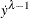, where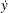is the geometric mean of the variable to be transformed. This form of the Box-Cox transformation essentially converts the transformation back to original units, and hence it permits direct comparison of the residual sums of squares for models with different power parameters.

LAMBDA=number-list
LAM=number-list

specifies a list of Box-Cox power parameters. The default is LAMBDA=–3 TO 3 BY 0.25. PROC TRANSREG tries each power parameter in the list and picks the best one. However, when the CONVENIENT t-option is specified, PROC TRANSREG chooses a convenient value from the confidence interval instead of the optimal value. For example, if the optimal power parameter is 0.25 and 0.0 is in the confidence interval but not 1.0, then the convenient power parameter 0.0 (log transformation) is chosen instead of the more optimal parameter 0.25. See the CLL= t-option for more information about its usage.

Other t-options

AFTER
AFT

requests that certain operations occur after the expansion. This t-option affects the NKNOTS= t-option when the SPLINE or MSPLINE transformation is crossed with a CLASS specification. For example, if the original spline variable (1 2 3 4 5 6 7 8 9) is expanded into the three variables (1 2 3 0 0 0 0 0 0), (0 0 0 4 5 6 0 0 0), and (0 0 0 0 0 0 7 8 9), then, by default, NKNOTS=1 would use the overall median of 5 as the knot for all three variables. When you specify the AFTER t-option, the knots for the three variables are 2, 5, and 8. Note that the structural zeros are ignored when the internal knot list is created, but they are not ignored for the exterior knots.

You can also specify the AFTER t-option with the RANK, SMOOTH, and PBSPLINE transformations. The following specifications compute ranks and smooth transformations within groups, after crossing, ignoring the structural zeros:

class(x / zero=none) | rank(z / after)
class(x / zero=none) | smooth(z / after)

CENTER
CEN

centers the variables before the analysis begins (in contrast to the TSTANDARD=CENTER option, which centers after the analysis ends). The CENTER t-option can be used instead of running PROC STANDARD before PROC TRANSREG (see the section Centering). When the KNOTS= t-option is specified with CENTER, the knots apply to the original variable, not to the centered variable. PROC TRANSREG centers the knots.

NAME=(variable-list)
NAM=(variable-list)

renames variables as they are used in the MODEL statement. This t-option lets you use a variable more than once.

For example, if x is a character variable, then the following step stores both the original character variable x and a numeric variable xc that contains category numbers in the OUT= data set:

proc transreg data=a;
model identity(y) = opscore(x / name=(xc));
output;
id x;
run;


With the CLASS and IDENTITY transformations, which can contain interaction effects, the first name applies to the first variable in the specification, the second name applies to the next variable that was not previously mentioned, and so on. For example, identity(a a * b b b * c c / name=(g h i)) specifies that the new name for a is g, for b is h, and for c is i. The same assignment is used for the (not useful) specification identity(a a b b c c / name=(g h i)). For all transforms other than CLASS and IDENTITY (all those in which interactions are not supported), repeated variables are not handled specially. For example, spline(a a b b c c / name=(a g b h c i)) creates six variables: a copy of a named a, another copy of a named g, a copy of b named b, another copy of b named h, a copy of c named c, and another copy of c named i.

REFLECT
REF

reflects the transformation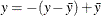after the iterations are completed and before the final standardization and results calculations. This t-option is particularly useful with the dependent variable in a conjoint analysis. When the dependent variable consists of ranks with the most preferred combination assigned 1.0, the REFLECT t-option reflects the transformation so that positive utilities mean high preference. (See Example 101.4.)

TSTANDARD=CENTER | NOMISS | ORIGINAL | Z
TST=CEN | NOM | ORI | Z

specifies the standardization of the transformed variables for the hypothesis tests and in the OUT= data set (see the section Centering). By default, TSTANDARD=ORIGINAL. When you specify TSTANDARD= as an a-option or an o-option, it determines the default standardization for all variables. When you specify TSTANDARD= as a t-option, it overrides the default standardization only for selected variables. You can specify a different TSTANDARD= value for each transformation. For example, to perform a redundancy analysis with standardized dependent variables, specify the following:

model identity(y1-y4 / tstandard=z) = identity(x1-x10);

Z

centers and standardizes the variables to variance one before the analysis begins (in contrast to the TSTANDARD=Z option, which standardizes after the analysis ends). The Z t-option can be used instead of running PROC STANDARD before PROC TRANSREG (see the section Centering). When the KNOTS= t-option is specified with Z, the knots apply to the original variable, not to the standardized variable. PROC TRANSREG standardizes the knots.

Algorithm Options (a-options)

This section discusses the options that can appear in the PROC TRANSREG or MODEL statement as a-options. They are listed after the entire model specification and after a slash. Here is an example:

proc transreg;
model spline(y / nknots=3)=log(x1 x2 / parameter=2)
/ nomiss maxiter=50;
output;
run;


In the preceding statements, NOMISS and MAXITER= are a-options. (SPLINE and LOG are transforms, and NKNOTS= and PARAMETER= are t-options.) The statements find a spline transformation with 3 knots on y and a base 2 logarithmic transformation on x1 and x2. The NOMISS a-option excludes all observations with missing values, and the MAXITER= a-option specifies the maximum number of iterations.

Table 101.4 summarizes the a-options available in the PROC TRANSREG or MODEL statement.

Table 101.4: Options Available in the PROC TRANSREG or MODEL Statement

Option

Description

Input Control

Restarts iterations

Specifies input observation type

Method and Iterations

Specifies minimum criterion change

Specifies minimum data change

Specifies maximum number of iterations

Specifies iterative algorithm

Specifies number of canonical variables

Specifies no restrictions on smoothing models

Specifies singularity criterion

Attempts direct solution instead of iteration

Missing Data Handling

Fits each model individually (METHOD=MORALS)

Includes monotone special missing values

Excludes observations with missing values

Unties special missing values

Intercept and CLASS Variables

Specifies CLASS coded variable name prefix

Specifies CLASS coded variable label prefix

Specifies no intercept or centering

Specifies order of CLASS variable levels

Controls output of reference levels

Specifies CLASS coded variable label separators

Control Displayed Output

Specifies confidence limits alpha

Displays parameter estimate confidence limits

Displays model specification details

Displays iteration histories

Suppresses displayed output

Prints the Box-Cox log likelihood table

Displays the R square

Suppresses the iteration histories

Displays regression results

Displays ANOVA table

Shortens transformed variable labels

Displays conjoint part-worth utilities

Standardization

Does not zero constant variables

Specifies transformation standardization

The following list provides details about these a-options. The a-options are available in the PROC TRANSREG or MODEL statement.

creates an additive model by multiplying the values of each independent variable (after the TSTANDARD= standardization) by that variable’s corresponding multiple regression coefficient. This process scales the independent variables so that the predicted-values variable for the final dependent variable is simply the sum of the final independent variables. An additive model is a univariate multiple regression model. As a result, the ADDITIVE a-option is not valid if METHOD=CANALS, or if METHOD=REDUNDANCY or METHOD=UNIVARIATE with more than one dependent variable.

ALPHA=number
ALP=number

specifies the level of significance for all of the confidence limits. By default, ALPHA=0.05.

CCONVERGE=n
CCO=n

specifies the minimum change in the criterion being optimized (squared multiple correlation for METHOD=MORALS and METHOD=UNIVARIATE, average squared multiple correlation for METHOD=REDUNDANCY, average squared canonical correlation for METHOD=CANALS) that is required to continue iterating. By default, CCONVERGE=0.0.

CL

requests confidence limits on the parameter estimates in the displayed output.

CONVERGE=n
CON=n

specifies the minimum average absolute change in standardized variable scores that is required to continue iterating. By default, CONVERGE=0.00001. Average change is computed over only those variables that can be transformed by the iterations; that is, all LINEAR, OPSCORE, MONOTONE, UNTIE, SPLINE, MSPLINE, and SSPLINE variables and nonoptimal transformation variables with missing values.

CPREFIX=n
CPR=n

specifies the number of first characters of a CLASS expansion variable’s name to use in constructing names for coded variables. Coded variable names are constructed from the first n characters of the CLASS expansion variable’s name and the first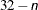characters of the formatted CLASS expansion variable’s value. For example, if the variable ClassVariable has values 1, 2, and 3, then, by default, the coded variables are named ClassVariable1, ClassVariable2, and ClassVariable3. However, with CPREFIX=5, the coded variables are named Class1, Class2, and Class3. When CPREFIX=0, coded variable names are created entirely from the CLASS expansion variable’s formatted values. Valid values range from –1 to 31, where –1 indicates the default calculation and 0 to 31 are the number of prefix characters to use. The default, –1, sets n to 32 – min(32, max(2, fl)), where fl is the format length. When you specify CPREFIX= as an a-option or an o-option, it specifies the default for all CLASS variables. When you specify CPREFIX= as a t-option, it overrides the default only for selected variables.

DETAIL
DET

reports on details of the model specification. For example, it reports the knots and coefficients for splines, reference levels for CLASS variables, Box-Cox results, the smoothing parameter, and so on. The DETAIL option can take two optional suboptions, NOCOEFFICIENTS and NOKNOTS (or NOC and NOK). To suppress knots from the details listing, specify DETAIL(NOKNOTS). To suppress coefficients from the details listing, specify DETAIL(NOCOEFFICIENTS). To suppress both knots and coefficients from the details listing, specify DETAIL(NOKNOTS NOCOEFFICIENTS).

SOLVE
SOL
DUMMY
DUM

provides a canonical initialization. When there are no monotonicity constraints, when there is at most one canonical variable in each set, and when there is enough available memory, PROC TRANSREG (with the SOLVE a-option) can usually directly solve for the optimal solution in only one iteration. The initialization iteration is number 0, which is slower and uses more memory than other iterations. However, for some models, specifying the SOLVE a-option can greatly decrease the amount of time required to find the optimal transformations. During iteration 0, each variable is replaced by an expanded variable and the model is fit to the larger, expanded set of variables. For example, an OPSCORE variable is expanded into coded (or dummy) variables, as if CLASS were specified, and a SPLINE variable is expanded into a B-spline basis, as if BSPLINE were specified. Then for each expanded variable, the results of iteration zero are constructed by multiplying the expanded basis times the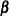subvector to get the optimal transformation. This a-option can be useful even in models where a direct solution is not possible, because it provides good initial transformations of all the variables.

HISTORY
HIS

displays the iteration histories even when the NOPRINT a-option is specified.

INDIVIDUAL
IND

fits each model for each dependent variable individually. This means, for example, that when INDIVIDUAL is specified, missing values in one dependent variable will not cause that observation to be deleted for the other models with the other dependent variables. In contrast, by default, missing values in any variable in any model can cause the observation to be deleted for all models. The INDIVIDUAL a-option can be specified only with METHOD=MORALS.

This a-option also affects the order of the output. By default, the number of observations table is printed once at the beginning of the output. With INDIVIDUAL, a number of observations table appears for each model.

LPREFIX=n
LPR=n

specifies the number of first characters of a CLASS expansion variable’s label (or name if no label is specified) to use in constructing labels for coded variables. Coded variable labels are constructed from the first n characters of the CLASS expansion variable’s name and the first 127 – n characters of the formatted CLASS expansion variable’s value. Valid values range from –1 to 127. Values of 0 to 127 specify the number of name or label characters to use. The default is –1, which specifies that PROC TRANSREG should pick a value depending on the length of the prefix and the formatted class value. When you specify LPREFIX= as an a-option or an o-option, it determines the default for all CLASS variables. When you specify LPREFIX= as a t-option, it overrides the default only for selected variables.

MAXITER=n
MAX=n

specifies the maximum number of iterations (see the section Controlling the Number of Iterations). By default, MAXITER=30. You can specify MAXITER=0 to save time when no transformations are requested.

METHOD=CANALS | MORALS | REDUNDANCY | UNIVARIATE
MET=CAN | MOR | RED | UNI

specifies the iterative algorithm. By default, METHOD=UNIVARIATE, unless you specify options that cannot be handled by the UNIVARIATE algorithm. Specifically, the default is METHOD=MORALS for the following situations:

CANALS

specifies canonical correlation with alternating least squares. This jointly transforms all dependent and independent variables to maximize the average of the first n squared canonical correlations, where n is the value of the NCAN= a-option.

MORALS

specifies multiple optimal regression with alternating least squares. This transforms each dependent variable, along with the set of independent variables, to maximize the squared multiple correlation.

REDUNDANCY

jointly transforms all dependent and independent variables to maximize the average of the squared multiple correlations (see the section Redundancy Analysis).

UNIVARIATE

transforms each dependent variable to maximize the squared multiple correlation, while the independent variables are not transformed.

MONOTONE=two-letters
MON=two-letters

specifies the first and last special missing value in the list of those special missing values to be estimated with within-variable order and category constraints. By default, there are no order constraints on missing value estimates. The two-letters value must consist of two letters in alphabetical order. For example, MONOTONE=DF means that the estimate of .D must be less than or equal to the estimate of .E, which must be less than or equal to the estimate of .F; no order constraints are placed on estimates of ._, .A through .C, and .G through .Z. For details, see the section Missing Values.

NCAN=n
NCA=n

specifies the number of canonical variables to use in the METHOD=CANALS algorithm. By default, NCAN=1. The value of the NCAN= a-option must be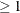.

When canonical coefficients and coordinates are included in the OUT= data set, the NCAN= a-option also controls the number of rows of the canonical coefficient matrices in the data set. If you specify an NCAN= value larger than the minimum of the number of dependent variables and the number of independent variables, PROC TRANSREG displays a warning and sets the NCAN= a-option to the maximum value.

NOINT
NOI

omits the intercept from the OUT= data set and suppresses centering of data. You cannot specify the NOINT a-option with iterative transformations since there is no provision for optimal scaling without an intercept. The NOINT a-option can be specified only when there is no implicit intercept and when all of the data in a BY group absolutely will not change during the iterations.

NOMISS
NOM

excludes all observations with missing values from the analysis, but does not exclude them from the OUT= data set. If you omit the NOMISS a-option, PROC TRANSREG simultaneously computes the optimal transformations of the nonmissing values and estimates the missing values that minimize squared error. For details, see the section Missing Values.

Casewise deletion of observations with missing values occurs when the NOMISS a-option is specified, when there are missing values in expansions, when there are missing values in METHOD=UNIVARIATE independent variables, when there are weights less than or equal to 0, or when there are frequencies less than 1. Excluded observations are output with a blank value for the _TYPE_ variable, and they have a weight of 0. They do not contribute to the analysis but are scored and transformed as supplementary or passive observations.

NOPRINT
NOP

suppresses the display of all output unless you specify the HISTORY a-option. The NOPRINT a-option without the HISTORY a-option disables the Output Delivery System (ODS), including ODS Graphics, for the duration of the procedure run. The NOPRINT a-option with the HISTORY a-option disables all output except the iteration history, again including ODS Graphics, for the duration of the procedure run. For more information, see Chapter 20: Using the Output Delivery System.

NOZEROCONSTANT
NOZERO
NOZ

specifies that constant variables are expected and should not be zeroed. By default, constant variables are zeroed. This option is useful when PROC TRANSREG is used to code experimental designs for discrete choice models (see the section Discrete Choice Experiments: DESIGN, NORESTORE, NOZERO). When these designs are very large, it might be more efficient to use the DESIGN=n a-option. It might be that attributes are constant within a block of n observations, so you need to specify the NOZEROCONSTANT a-option to get the correct results. You can specify this option in the PROC TRANSREG, MODEL, and OUTPUT statements.

NSR

specifies that no restrictions are placed on the use of SMOOTH and SSPLINE and the ordinary least squares is used to find the coefficients and predicted values. By default, only certain types of models can be specified with SMOOTH and ordinary least squares is not used to find the coefficients and predicted values. See the section Smoothing Splines Changes and Enhancements for more information about the NSR option and smooth transformations.

ORDER=DATA | FREQ | FORMATTED | INTERNAL
ORD=DAT | FRE | FOR | INT

specifies the order in which the CLASS variable levels are to be reported. The default is ORDER=INTERNAL. For ORDER=FORMATTED and ORDER=INTERNAL, the sort order is machine dependent. When you specify ORDER= as an a-option or an o-option, it determines the default ordering for all CLASS variables. When you specify ORDER= as a t-option, it overrides the default ordering only for selected variables.

DATA

sorts by order of appearance in the input data set.

FORMATTED

sorts by formatted value.

FREQ

sorts by descending frequency count; levels with the most observations appear first.

INTERNAL

sorts by unformatted value.

PBOXCOXTABLE
PBO

prints the Box-Cox table with the log likelihood displayed as a function of lambda. The important information in this table is displayed in the Box-Cox plot, so when ODS Graphics is enabled and the plot is produced, the table is not produced by default. When ODS Graphics is not enabled or when the plot is not produced, the table is produced by default. Specify the PBOXCOXTABLE option if you want to see the table in addition to the plot.

REFERENCE=NONE | MISSING | ZERO
REF=NON | MIS | ZER

specifies how reference levels of CLASS variables are to be treated. The options are REFERENCE=NONE, the default, in which reference levels are suppressed; REFERENCE=MISSING, in which reference levels are displayed and output with missing values; and REFERENCE=ZERO, in which reference levels are displayed and output with zeros. You can specify the REFERENCE= option in the PROC TRANSREG, MODEL, or OUTPUT statement, and you can specify it independently for the OUT= data set and the displayed output. When you specify it in only one statement, it sets the option for both the displayed output and the OUT= data set.

REITERATE
REI

enables PROC TRANSREG to use previous transformations as starting points. The REITERATE a-option affects only variables that are iteratively transformed (specified as LINEAR, OPSCORE, MONOTONE, UNTIE, SPLINE, MSPLINE, and SSPLINE). For iterative transformations, the REITERATE a-option requests a search in the input data set for a variable that consists of the value of the TDPREFIX= or TIPREFIX= o-option followed by the original variable name. If such a variable is found, it is used to provide the initial values for the first iteration. The final transformation is a member of the transformation family defined by the original variable, not the transformation family defined by the initialization variable. See the section Using the REITERATE Algorithm Option for more information about the REITERATE option.

RSQUARE
RSQ

prints a table with only the model R square.

SEPARATORS=’string-1’ <’string-2’>
SEP=’string-1’ <’string-2’>

specifies separators for creating CLASS expansion variable labels. By default, SEPARATORS=’ ’ ’ * ’ (blank and blank asterisk blank). The first value is used to separate variable names and values in interactions. The second value is used to separate interaction components. For example, the label for the coded variable for the A=1 and B=2 cell is, by default, ’A 1 * B 2’. If SEPARATORS=’=’ ’x’ is specified, then the label is ’A=1xB=2’. When you specify SEPARATORS= as an a-option or an o-option, it determines the default separators for all CLASS variables. When you specify SEPARATORS= as a t-option, it overrides the default only for selected variables.

SHORT
SHO

suppresses the iteration histories.

SINGULAR=n
SIN=n

specifies the largest value within rounding error of zero. By default, SINGULAR=1E–12. PROC TRANSREG uses the value of the SINGULAR= a-option for checking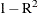when constructing full-rank matrices of predictor variables, checking denominators before dividing, and so on. PROC TRANSREG computes the regression coefficients by sweeping with rational pivoting.

SS2

produces a regression table based on Type II sums of squares. Tests of the contribution of each transformation to the overall model are displayed and output to the OUTTEST= data set when you specify the OUTTEST= option. When you specify the SS2 a-option, the TEST a-option is automatically specified for you. See the section Hypothesis Tests for more information about the TEST and SS2 options. You can suppress the variable labels in the regression tables by specifying the NOLABEL option in the OPTIONS statement.

TEST
TES

generates an ANOVA table. PROC TRANSREG tests the null hypothesis that the vector of scoring coefficients for all of the transformations is zero. See the section Hypothesis Tests for more information about the TEST option.

TSUFFIX=n
TSU=n

specifies the number of characters in Transformation to append to variable labels for transformed variables. By default, all characters are used.

TSTANDARD=CENTER | NOMISS | ORIGINAL | Z
TST=CEN | NOM | ORI | Z

specifies the standardization of the transformed variables for the hypothesis tests and in the OUT= data set. By default, TSTANDARD=ORIGINAL. When you specify TSTANDARD= as an a-option or an o-option, it determines the default standardization for all variables. When you specify TSTANDARD= as a t-option, it overrides the default standardization only for selected variables.

CENTER

centers the output variables to mean zero, but the variances are the same as the variances of the input variables.

NOMISS

sets the means and variances of the transformed variables in the OUT= data set, computed over all output values that correspond to nonmissing values in the input data set, to the means and variances computed from the nonmissing observations of the original variables. The TSTANDARD=NOMISS specification is useful with missing data. When a variable is linearly transformed, the final variable contains the original nonmissing values and the missing value estimates. In other words, the nonmissing values are unchanged. If your data have no missing values, TSTANDARD=NOMISS and TSTANDARD=ORIGINAL produce the same results.

ORIGINAL

sets the means and variances of the transformed variables to the means and variances of the original variables. This is the default.

Z

standardizes the variables to mean zero, variance one.

The final standardization is affected by other options. If you also specify the ADDITIVE a-option, the TSTANDARD= option specifies an intermediate step in computing the final means and variances. The final independent variables, along with their means and standard deviations, are scaled by the regression coefficients, creating an additive model with all coefficients equal to one.

For nonoptimal variable transformations, the means and variances of the original variables are actually the means and variances of the nonlinearly transformed variables, unless you specify the ORIGINAL nonoptimal t-option in the MODEL statement. For example, if a variable x with no missing values is specified as LOG, then, by default, the final transformation of x is simply the log of x, not the log of x standardized to the mean of x and variance of x.

TYPE=’text’|name
TYP=’text’|name

specifies the valid value for the _TYPE_ variable in the input data set. If PROC TRANSREG finds an input _TYPE_ variable, it uses only observations with a _TYPE_ value that matches the TYPE= value. This enables a PROC TRANSREG OUT= data set containing coefficients to be used as input to PROC TRANSREG without requiring a WHERE statement to exclude the coefficients. If a _TYPE_ variable is not in the data set, all observations are used. The default is TYPE=’SCORE’, so if you do not specify the TYPE= a-option, only observations with _TYPE_=’SCORE’ are used. Do not confuse this a-option with the data set TYPE= option. The DATA= data set must be an ordinary SAS data set.

PROC TRANSREG displays a note when it reads observations with blank values of _TYPE_, but it does not automatically exclude those observations. Data sets created by the TRANSREG and PRINQUAL procedures have blank _TYPE_ values for those observations that were excluded from the analysis due to nonpositive weights, nonpositive frequencies, or missing data. When these observations are read again, they are excluded for the same reason that they were excluded from their original analysis, not because their _TYPE_ value is blank.

UNTIE=two-letters
UNT=two-letters

specifies the first and last special missing values in the list of those special missing values that are to be estimated with within-variable order constraints but no category constraints. The two-letters value must consist of two letters in alphabetical order. By default, there are category constraints but no order constraints on special missing value estimates. For details, see the sections Missing Values and Optimal Scaling.

UTILITIES
UTI

produces a table of the part-worth utilities from a conjoint analysis. Utilities, their standard errors, and the relative importance of each factor are displayed and output to the OUTTEST= data set when you specify the OUTTEST= option. When you specify the UTILITIES a-option, the TEST a-option is automatically specified for you. See Example 101.4 and Example 101.5 for more information about conjoint analysis.